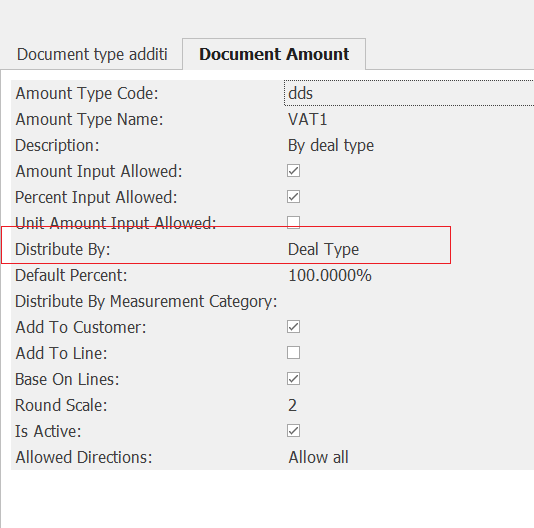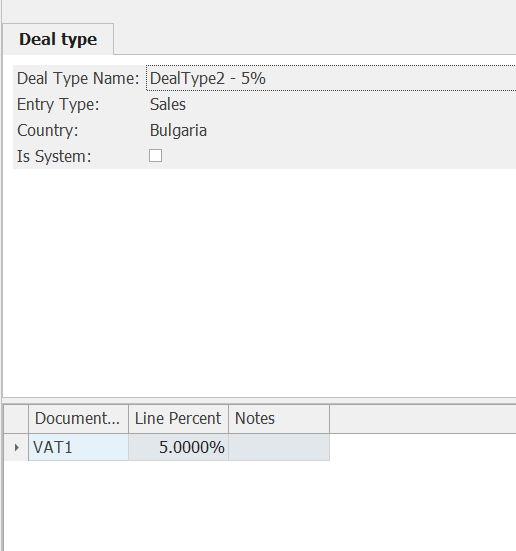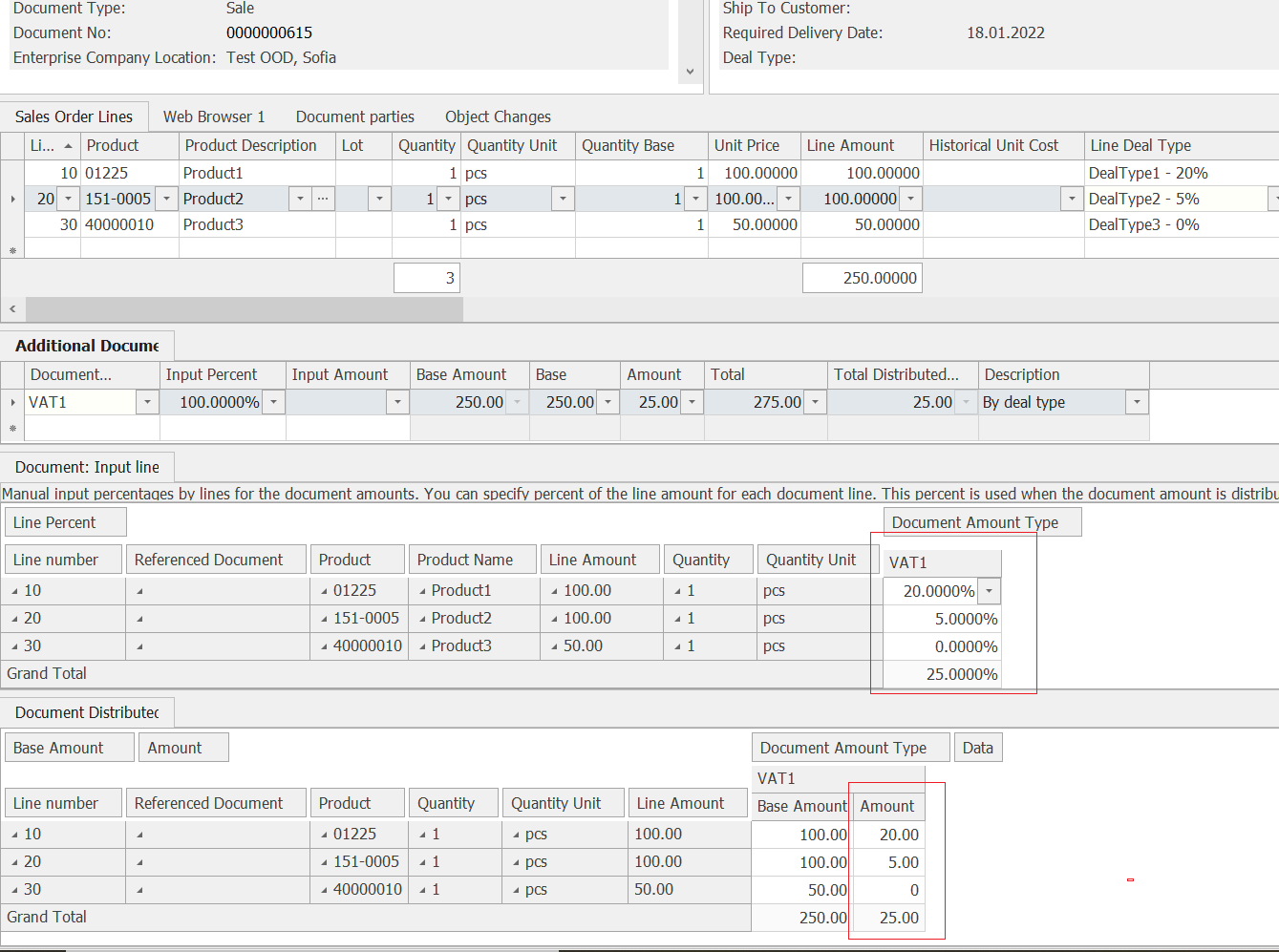# New type of distribution of additional amounts "By Deal Type" (v.22)

In order to accommodate the use of multiple VAT rates across the lines of a single document, a new type of distribution of additional amounts is being introduced - "By Deal Type".For this purpose, the definition of the deal type now allows specifying a percent for the additional amount.When using this type of distribution, the percentages specified in the deal type definition are copied to the additional amounts per line in the documents.The additional amounts are calculated for each line using the following formula:

Distributed Line Amount = Line Base Amount * Line Percent * Input Percent

When using input percentages per line, it is typically necessary to set the Input Percent in the document to 100%. This is because this percentage is multiplied by the line percentage before being applied to the line base.